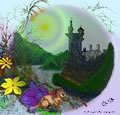# Natural numbers (whole numbers), integers, rationals, real numbers, complex numbers and their operations.

Overview

## Number definitions

• Natural numbers known as counting numbers and whole numbers. They are 1, 2, 3, 4, ... and sometimes include 0.
• Integers positive and negative whole numbers. Numbers that can be written without a fraction. They are ... -4, -3, -2, -1, 0, 1, 2, 3, 4, ...
• Rational numbers are numbers that can be represented as a fraction (N/D) of two integers. N is the a numerator and D is the denominator. D is always defined as not zero (0). If D is equal to one (1), then it is an integer. Therefore, every integer is a rational number. The set of all rational numbers is usually represented as Q. A decimal number is a rational number if it terminates. That is it has a finite number of digits or repeats a finite sequence of digits (.125125...). These statements hold true for any integer base number (binary, hexadecimal).
• Irrational numbers are numbers such as √2 (1.41421356…, π (3.14159265…. Numbers that do not terminate as a decimal number.
• Real numbers include rational numbers (integers, fractions) and irrational numbers. Real numbers can be represented as a point on a number line. Where points represent equally spaced integers.Photo source: Phrood [Public domain], via Wikimedia Commons

## Instructional Activities and Teacher tools

### Activities and Instructional Suggestions for number sense

Number relationships --->>> see patterns and algebra

### Manipulatives for students to use for number value

Cards for students to sort

Die roll ---

Children confuse numbers, lines, and spaces on number lines making their use questionable for children below second grade.

## Place value

### Really big numbers and infinity

• Olber's Paradox - if the universe is infinite in time and space, stars should occupy every point in space and fill the night sky with light.
• How many grains of sand in the Universe? Archimedes' proof in The Sand Reckoner.

## Basic Operations

### Fractional numbers

Division problems represented with squares and rectangles

• Sample problems: 1/2 ÷ 1/2, 1/2 ÷ 1/4 , 1/2 ÷ 3/4, 1/2 ÷ 3/5 (thanks Brant),

### Integers

Addition and subtraction of positive and negative integers might be conceptualized by representing and controling two properties: position on a number line and direction of body.

The number line can have two halves with the same numbers being both positive and negative. You have two choices for each number + or - as a reference to position. If the number has a value of 4, it could be represented as a positive four or negative four.

You can represent those two numbers by standing on either +4 or -4. Secondly when you are standing on a number line you can face one of two direction, since the number line is bidirectional, you might be facing left or right, forward or backward, in a positive direction or negative direction. or if it is thought of as a thermometer, you can walk hotter or colder.

Problems can be posed as:
Find a starting point, say -3 and if you add a +3, face warmer and walk forward 3.
Find a starting point, say -3 and if + -3, then face warmer and walk backward.
Find a starting point, say -3 and if - - 3, then face colder and walk backward.
Find a starting point, say -3 and if - +3, then face colder and walk forward.

Again both are important and a concrete model can be used to describe a procedural rule, as demonstrated as a plus and a plus are plus, a plus and a minus are a minus, and a minus and a minus are a plus.

Another idea is to record a video with motion. People walking or running forward and backward. They might display signs with forward and backward as they do so. However, it probably will be obvious which direction they moving when it is recorded. When the video is made, then each can be viewed with the movie play forward and backward. A chart of the different results can be made (forward, forward, results in forward; forward, backward, results in backward; backward, forward results in backward; backward, backward results in forward.

After several concrete experience have been experienced explore the this challenge.
How many different ways can two numbers be represented with different a sign and an operation (-2 ++3; -2 -+3; -2 --3; -2 +-3) (2 ++3; 2 -+3; 2 --3; 2 +-3).